American Journal of Engineering and Technology Management
Volume 1, Issue 4, December 2016, Pages: 59-64

Genetic Algorithms Techniques Based Optimal PID Tuning For Speed Control of DC Motor

Santosh Kumar Suman, Vinod Kumar Giri

Departement of Electrical Engineering, Madan Mohan Malaviya University of Technology, Gorakhpur, India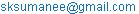(S. K. Suman)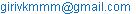(V. K. Giri)

Santosh Kumar Suman, Vinod Kumar Giri. Genetic Algorithms Techniques Based Optimal PID Tuning For Speed Control of DC Motor. American Journal of Engineering and Technology Management. Vol. 1, No. 4, 2016, pp. 59-64. doi: 10.11648/j.ajetm.20160104.12

Received: August 29, 2016; Accepted: September 26, 2016; Published: November 7, 2016

Abstract: Plan of this paper a investigation and implementation of controllers such as PID controller and G.A based PID for speed control of DC motor. Simulation results have established that the use of PID and GA-PID. A DC motor is significant for a good dynamic, reliable behavior of the DC motor, a great speed tracking with lowest overshoot, gives enhanced performance and high strength than those obtained by use of the other controller. The DC motor is broadly used in many applications like steel mills, electric trains, cranes and much more. In this dissertation a separately excited dc motor using MATLAB modeling has been outlined whose velocity might be examined utilizing the Proportional, Integral, Derivative (KP, KI, KD) addition of the PID controller. In this paper is to analyze the execution of Optimization techniques viz. The Genetic Algorithm (GA) for improve PID controllers parameters for speed control of DC motor and list their points of interest over the traditional tuning strategies. The output speed error and its derivative as feedback damping signals. In this we have create three objective function with help of the MATLAB coding m-file, but third objective function is a novel creation for system which gives the better result than conventional objective function.aim of this paper compared all conventional method to proposed genetic algorithm tuning techniques and finds optimum results such as peak time, overshoot and transient response

Keyword: DC motor, PID Controller, Genetic Algorithm (GA), IAE, MSE

Contents

1. Introduction

Nowadays the DC motor is most important motor because of its dynamic and reliable behavior. The main challenge of dc motor to control of its speed for performing a desired tasked motor is high performance motor drive system are its very important in engineering and industrials as well as other purpose applications such as electric trains, steel rolling mills, and robotics. usually, a high performance motor drives system have good dynamic response which perform speed command tracking and load regulating response [1, 2]. DC motor drives cover the simplicity, no difficulty of application, flexibility, high consistency and favorable cost.

Hence DC motor drive is the important part of industrial applications, home appliances and robot

Manipulators where position controls of motor are required and speed. DC motor drives consist of less complexity, with a single power conversion from AC to DC. DC motor is to convert into electrical energy to mechanical energy called rotational. Again the speed torque characteristics of DC motors are much more superior to that of AC motors .

DC motor drives provide an excellent control of speed for acceleration and deceleration and normally less expensive for most horsepower ratings. Therefore DC motors have been used traditionally for adjustable speed machines and a wide range of speed control systems has evolved for this purpose. In these applications, the motor should be precisely controlled to give the desired performance. The controllers that desired to control the speed of DC motor are of several conventional and numeric types e.g. Proportional Integral (PI), Proportional Integral Derivative (PID), Genetic algorithms or combinations of them e.g. GA-PID, . A PID (Proportional Integral Derivative) controller is a common feedback loop component in industrial control applications. PID controller has been widely used in the industry because of its simple structure and robust performance in a wide range of operating condition . Over the years, several typical methods have been proposed for the tuning of PID controllers. Such as Ziegler-Nichols, frequency domain method and Time domain method. With the development of the computer technology and Artificial Intelligence (AI).In the 70s later century, the GA have developed very much, which has parallel search techniques and emulate natural genetic operation. It has widely been applied to tune PID controllers . PID stands for Proportion-Integral-Differential control. Each of these, the P, the I and the D are terms in a control algorithm, and each has a special purpose. Conventional PID controller has a simple structure and can provide satisfactory performance for many control problems. For process control systems, in particular, more than 95% of the controllers used are of PID type . This is because it is easily applicable to field use, and also easily adjustable .The most important trouble in applying a classical control procedure (PI, PD, PID) in a speed controller are the effects of non-linearity in a DC motor. The nonlinear characteristics of a DC motor such as saturation and friction could degrade the performance of conventional controllers . However in this chapter, the selections of optimal PID controller parameter are introduced and useful based on genetic algorithms technique. Thus it is likely to discover hereditarily the optimal controller parameter Kp, Ki, and Kd through the control of minimizing absolute or square error signal to accomplish system reliability furthermore to enhance system performance. Although in comeback be in command of systems the for the most part significant obligation is the complete system.

The target of this paper is to look at the implementation of Genetic Algorithm (GA) for perfect tuning of PID controllers parameters. We have finally different tuning techniques like P, PI, PID through the simulate DC motor then we have getting the different result. The Genetic Algorithms were utilized to overview the ideal PID controller extension values where execution records, IAE were utilized as far as possible. It was most likely settled that the Integral of Absolute Magnitude of the error (IAE) execution foundation delivers the best PID controller when contrasted and other execution paradigm This paper is composed as takes after: system modeling of DC motor is displayed in Section II, PID controller brief describe in section III, brief prologue to genetic algorithm is talked about in Section IV, main work of this paper describe in section V as a tuned methodology and last two Section VI and VII individually describe simulation result and conclusion of this paper speed control of dc motor.

2. Separately Excited DC Motor

2.1. Physical System

The SEDC motor drive system through armature control and the voltage apply to armature of the motor is familiar without realignment the voltage functional to the field. Figure. 1. shows a separately excited DC motor equivalent model (SEDC). It is assemble of the circuit model of dc motor using MATLAB/Simulink as shown in Figure. 2. In this a special case through the supply provided a separately to armature winding and field winding. The main a different or distinct form in these types of dc motor is with the main purpose of the field winding in does not flow the armature current because, the field winding is agitated from a separate external source of dc current. DC motors gives outstanding control of speed for motors require of their main parameters such as position, speed, acceleration etc . DC motor is a high performance motor drive. The dc motor drive is based on the principal, when a current carrying conductor is to be found in a magnetic fields, it experience a force which has a tendency to move. This is known as motoring action or rotating function, when magnetic field and electric field work together they produce a mechanical force.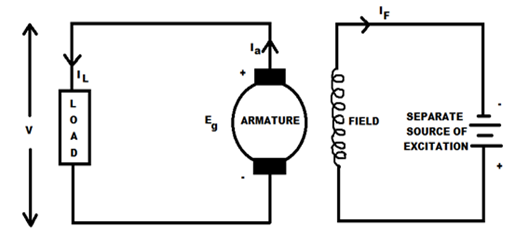Fig. 1. S E D C Motor.

The rotor and the shaft of Separately Excited DC motor are assumed to be rigid. Consider the following values for the physical parameters 

Table 1. DC motor of parameters.

 Parameters Value Armature inductance (Henry)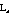= 0.1215H Armature resistance (ohm)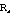= 11.2 Ω Rotor inertia (kg m2)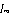= 0.02215 kgm2 Armature voltage (Volt)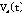= 240V Viscous friction coefficient (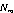s/rad)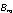= 0.002953s/rad Motor torque constant (Nm/A)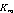= 1.28 Nm/A Back emf constant (V s/rad)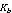= 1.28 V s/rad Speed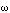= 1500rpm

2.2. Mathematical Model of DC Motor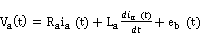(1)(2)

The motor torque, Tm, is related to the armature current, ia, by a constant factor Km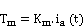(3)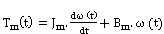(4)

Let us join the above equations (1) to (4) together and taking the Laplace transforms

Yields,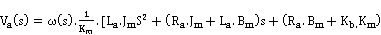(5)

After that the relation between rotor shaft speed and applied armature voltage is represented by

Transfer function: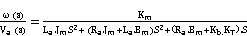(6)

And the transfer functions between shaft position and armature voltage at no-load are: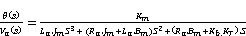(7)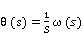(8)

Therefore, at this moment it is achievable to build the Simulink DC motor model, using the transfer functions (5) to (8) as shown in Fig. 2. Motor model was converted to a 2-in 4-out subsystem. Input ports are armature voltage and load torque (Tload) and the output ports are armature current, motor torque, angular shaft speed and position.

Where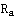= armature resistance (Ω- ohm).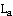= armature inductance (H-henry).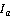= armature current (A).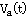= armature voltage (V).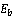= back emf (V).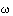= angular speed (rad/s).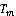= motor torque (N m).

θ = angular position of rotor shaft (rad).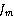= rotor inertia (kg m2).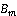= viscous friction coefficient (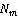s/rad).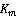= motor torque constant (Nm/A).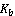= back emf constant (V s/rad).

3. Tuning of PID Controller

PID controllers have been commonly used for control systems. The most significant step for applying the PID controller is the tuning of its parameters. The tuning process requirements a lot of time and exertion. In the most terrible case, the bad tuning leads to a poor performance of the controlled system. The goal of the tuning process is to determine the PID controller parameters that satisfy the performance specifications of the controlled system, such as the rising time, the maximum overshot, the settling time and the steady state error. However, it is difficult to obtain the desirable values of these requirements simultaneously. As shown in Table 1, for example, larger values of proportional gain results in faster response while overshoot is increased. Therefore, an optimum tuning technique is of great importance.

4. PID Controller Design

In general, the Design Procedure for determining parameters of the PID controller is a trial and error approach. After obtaining a mathematical model of the control system and adjust the parameters of a compensator to improve system performance. The most time consuming part of such work is the checking of the system performance by analysis with each adjustment of the parameters.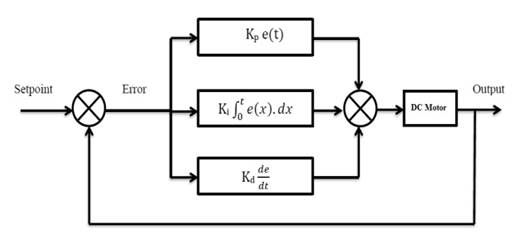Fig. 2. Essential block diagram of a PID controller.

General form of the Transfer function of a PID controller is given as,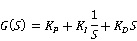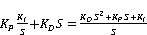=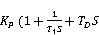)

Error, e (t) = Setpoint- Plant output

Where e = Error signal

Kp = Proportional Constant

Ki= Integral Constant

Kd = Derivative Constant

U (t) =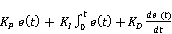The Tuning of a PID controller going to the tuning of its various parameters (P, I and D) to accomplish an optimized value of the required response. The fundamental needs of the output will be the strength, desired overshoot rise time and peak time.

4.1. PID Tuning Method

Ziegler and Nichols presented simple mathematical

Procedures to tune the parameters of the PID controller. These procedures have been accepted as a standard tuning method in the control systems. ZN method is applied on the plants whose step response of the form shown in Fig. 4. By drawing a tangent line at the inflection point of the step response of the plant, parameters K, L, and T (or a=KL/T) can be obtained.

Hence, the parameters of the PID controller can be calculated using Table 2.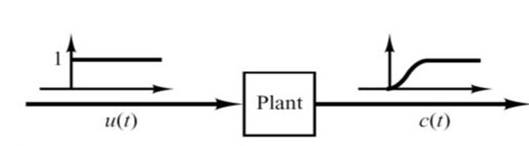Fig. 3. Mathematical of PID.

Table 2. PID Tuning Method.

 Controller type Controller Kp Ti Td P 1/a PI 0.9/a L/0.3 PID 1.2/a L/0.5 0.5L

4.2. Genetic Algorithm Tuning Method

Genetic Algorithm is a stochastic global search method that mimics the process of natural evolution. The genetic algorithm starts with no knowledge of the correct solution and depends entirely on responses from its environment and evolution operators (i.e. reproduction, crossover and mutation) to arrive at the best solution. By starting at several independent points and searching in parallel, the algorithm avoids local minima and converging to sub optimal solutions. In this way, GA has been shown to be capable of locating high performance areas in complex domains without experiencing the difficulties associated with high dimensionality, as may occur with gradient descend techniques.

In this paper, Genetic Algorithm approach is used for the following two purposes:

To determine the optimized value of PI controller parameters namely Kp, Ki and Kd

To reduce the error value between given higher order and obtained reduced order models.

In this paper, GA is used to determine the optimum values of the PID controller parameters that satisfy the required dynamic performance characteristics of the DC motor drive system. Fig. 5 shows the flowchart of GA based tuning of PID controller parameters. In the first, GA is initialized. Then, it creates an initial population of PID controller parameters. The population is generated randomly, covering the entire range of possible solutions. The population is composed of chromosomes. Each chromosome is a candidate solution to the problem. Fig. 6 shows the chromosome structure, in which the three parameters (Kp, Ki and Kd) are included. The chromosomes are applied in the DC drive plant and the dynamic performance characteristics of the plant are determined for each chromosome. Then, the fitness value for each chromosome is evaluated using the objective function. Based on the fitness values of the first generation, a group of best chromosomes is selected to create the next population. After selection, crossover and mutation are applied to this surviving population in order to improve the next generation.

The process continues until the termination criterion is achieved or the number of generations is reached to its maximum value.

5. Ga-based Optimization

The fitness function is the key to use the GA. The most essential stride in applying GA tuning strategy is to pick the target work that is utilized to assess the fitness value of every chromosome. In this dissertation, an objective functions are utilized and their execution is looked at. The first depends on integral of the absolute error (IAE), Integral of Squared Error and Mean of the Square of the Error index these report in objective function additionally plan through the MATLAB coding which is given in appendix.The parameters of GAs in this study are set as in Table 3. The GA advancement process based IAE index Fig. 6. For every case, the PID controller parameters are resolved. The objective function is given as:

IAE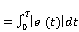ISEMSE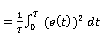the output response of the system is shown in Fig and we analyze the system for the previous parameters

Maximum overshoot, MP

Settling time,ts

Rise time, tr

the maximum overshoot, MP of the system is around zero. the settling time is about then we have go for studying the consequence of fuzzy logic controller for system and compare the PID based genetic algorithm

Table 3. Settings of GA parameters values.

 Parameter Value Lower bound [Kp Ki Kd] [0 0 0] Upper bound [Kp Ki Kd] [500 500 500] Populations 25 Generations 50 Population type Double vector Ranges of PID parameters 0-500 Crossover fraction 0.8 Mutation rate 0.01 Elite count 5 Selection function Tournament

6. Simulation Results and Discussion

The most important step in applying GA tuning method is to choose the objective function that is used to evaluate the fitness value of each chromosome. In this paper, three objective functions are used and their performances are compared. The first is based on integral of the absolute error (IAE) index, the second is based on mean of the squared error (MSE) index. The disentanglement or linearization of the non-direct system under thought must be performed by the traditional control approaches like P, PI, and PID since their development depends on straight system theory. Thus, these controllers don't any assurance for good execution . These controllers however are not prescribed for higher request and complex frameworks as they can bring about the system to end up flimsy. Subsequently, a more heuristic methodology is required. Using these controller parameters, the higher order system is tuned and the step response of the higher order system with GA based PI controller. The step response of the reduced order system with GA PI controller, The Step response of the reduced order system with GA -PID controller.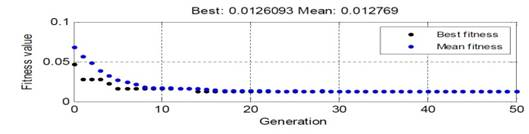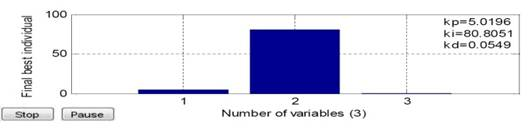Fig. 4. GA optimization process based IAE index.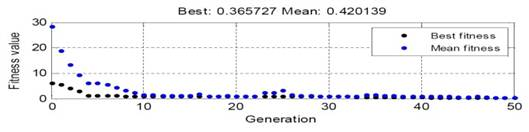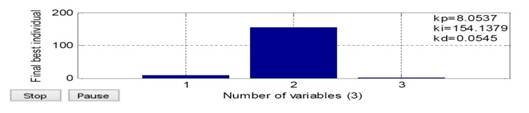Fig. 5. GA optimization process based ISE index.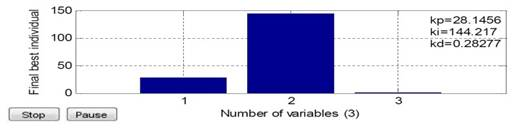Fig. 6. GA optimization process based MSE index.

From the recreation results In the above Table 4:it is inferred that by step reaction particulars utilizing Fuzzy controller The rise time of With PID controller is 0.104 sec and with FLC is 0.120sec. From these outcomes it can be see n that Fuzzy controllers have better steadiness, little overshoot and quick reaction. is superior to anything PID controller. Fuzzy controller results in less wavering at the control framework reaction. Less motion implies better controllability and less affectability to change in framework condition.

Table 4. Comparison of Results of Controllers Response.

 Tuning method Genetic Algorithm PARAMETERS P PI PID IAE objective function MSE objective function ISE objective function KP 14.7312 5.0196 8.0537 8.0537 KI 105.15 80.8051 154.1379 154.1379 KD 0.547 0.0549 0.0545 0.9545 Rise time (sec) 0.342 0.153 0.00622 0.0663 0.0499 0.0291 Settling time (sec) 1.08 0.543 0.0837 0.116 0.117 0.0952 Overshoot (%) 8.37 1.11 47.4% 0% 2.22% 8.33 Peak 0.773 0.216 1.41 1.02 0.995 1.08

7. Conclusion

In this paper we have discussed the speed control of DC motor Drive by different approach of controller. It is clear result that the ordinary PID controller is not getting the exact result but rather through the developmental calculation procedures to the ideal tuning of PID controller prompted an agreeable close circle reaction for the framework under thought. Examination of the outcomes as appeared in Table 4. This paper exhibits another tuning technique for speed control of DC motor utilizing genetic algorithm (GA) based The PID controller. Target of this paper of PID parameters upgrade through the genetic algorithm based distinctive target work, this tuning technique keeping in mind the end goal to accomplish least ascent time, settling time overshoot and steady state error but GA-ISE controller is gives the better results than all the controller the final results that gives more enhanced execution when contrasted with traditional PID controller for the considered system and thus, demonstrated the prevalence of the Genetic Algorithms. In this finally go to GA-PID best result than other controllers.

References

1. Yodyium tipsuwan, Mo-Yuen Chow "Fuzzy Logic Microcontroller Implementation for DC Motor Speed Control", IEEE Trans. syst. pp. 1271-1276, 1999.
2. Singari Pavan Kumar,Sande Krishna Veni, Y.B.Venugopal, and Y.S.Kishore Babu, A Neuro-Fuzzy based Speed Control of Separately Excited DC Motor, IEEE Transactions on Computational Intelligence and Communication Networks, pp. 93-98, 2010.
3. Boumedience Allaoua, Abdellah Laoufi,Brahim Gasbaoui, Abdelfatah Nasri and Abdessalam Abderrahmani, "Intelligent Controller Design for DC motor Speed control Based on Fuzzy Logic-Genetic Algorithms Optimization", Leonardo Journal of Science, vol. no. 13, pp. 90-102, July-December 2008.
4. Santosh Kumar Suman, Vinod Kumar Giri, "Genetic Algorithms: Basic Concepts and Real World Applications", International Journal of Electrical, Electronics and Computer Systems (IJEECS), Vol -3, Issue-12, 2015.
5. Ahemd EI-Bakly, A.Fouda, W. Sabry, "A Proposed DC Motor Sliding Mode Position Controller Design using Fuzzy Logic and PID Techniques" 13th International Conference on Aerospace Science &Avitation Technology, vol. no. 13, May 26-28, 2009.
6. JinKun Liu, Advanced PID control and Matlab simulation (second edition), Publishing house of electronics industry, Sept.2004.
7. B. Portens and A. H. Jones, "Genetic tuning of digital PID control,"Electronics Letters, vol. 28, no. 9, pp.834-844, 1992.
8. "PID Control," in The Control Handbook, W. S. Levine, Ed. Piscataway, NJ: IEEE Press, 1996, pp. 198–209.
9. Katsuhiko Ogata,"Modern Control Engineering" 4th edition Prentice Hall, (2002).
10. Santosh Kumar Suman and Vinod Kumar Giri,"Implementation of optimization and intelligent techniques for Speed Control of DC Motor -A Review", Karpagam Journal of Engineering Research (KJER),Vol- 5, Issue 1, pp-14-21,2016.
11. Sheroz Khan, Salami Femi Abdulazeez, Lawal Wahab Adetunji, AHM Zahirul Alam, Momoh Jimoh E. Salami, Shihab Ahmed Hameed, Aisha Hasan Abdalla and Mohd Rafiqul Islam, "Design and Implementation of an Optimal Fuzzy Logic Controller Using Genetic Algorithmic", Journal of Computer Science Malaysia, pp. 799-806, 2008.
12. Santosh Kumar Suman, Vinod Kumar Giri,"Investigation & implementation of speed control of DC Motor", JEE - Journal of Electrical Engineering,Vol-16,Issue-2,pp.315-322,2016.
13. Franz Rothlauf, "Representations for Genetic and Evolutionary Algorithms", Springer-Verlag Berlin Heidelberg, 2006.
14. Jin-Sung Kim, Jin-Hwan Kim, Ji-Mo Park, Sung-Man Park, Won- Yong Choe, Hoon Heo, Auto Tuning PID Controller based on Improved Genetic Algorithm for Reverse Osmosis Plant, World Academy of Science, Engineering and Technology, No. 23, 2008.
15. Y. K. Soni, R. Bhatt, BF-PSO optimized PID Controller design using ISE, IAE, IATE and MSE error criteria, International Journal of Advanced Research in Computer Engineering & Technology (IJARCET),Vol. 2, Issue 7, July 2013.

 Contents 1. 2. 2.1. 2.2. 3. 4. 4.1. 4.2. 5. 6. 7.
Article ToolsAbstractPDF(344K)